# Derivation of the DFT

## The theory and the praxis

The Discrete Fourier transform is defined as: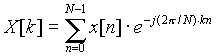It is derived from the continuous version of the Fourier series development: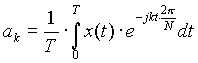It is not easy to see from the formulas what they mean.

## The story of sines

Where do sines come from? We did saw the circle drawing the sine, but... Most of the math before the 20th century was based or derived from or for physics.

If you bump an object and observe his response, it is very likely that the response or shaking will be very sine like. Because the sine has been observed in nature it is why the sine is called a natural function and the frequency of the sine is called the natural frequency. Looking from the practical point of view, it is very nice that man was able to find such a good mathematical model for that motion, namely the sine.

But most objects do not respond with only one sine frequency. This is where the Fourier transform can be used. Fourier offered a method to detect which frequencies of sines were present in the actual response of the object. His method can extract their frequency, phase and amplitude.

What about if the system does not have a sine function as his natural function? There was a lot of heat burned on that subject in the previous century. The conclusion was the following: It may be so, but with a sum of a sufficiently large number of different sines (the Fourier series) it is possible to approximate any desirable function.

## Finding the shapes

The Fourier found a way to model a complicated signal with a sum of sines that have different frequencies, amplitudes and phase. Let us try the same. In computer vision there is a widely accepted approach for finding the shapes on the picture, called Hough transform. The idea is simple. If we search for a straight line, that line has a mathematical form of: y = kx + n. We have two parameters: k and n. Now we form pairs of all possible values of k and n, draw the line and count the number of pixels that support that hypothesis. We save the intermediate results and at the end we apply a threshold to those results keeping only those pairs of parameters k and n that had a sufficiently large support.

If we can search for straight lines, why couldn't we search for sines? The sines have three parameters. The function is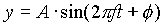The parameters are: A, f and phi. This approach works well, if we have only one sine that has to be found. If we have to find a function that is actually a sum of sines, the approach has to be modified.

## The orthogonality

One fundament of Linear algebra is orthogonality. Example:

Series 1: 1, 0, 0
Series 2: 0, 1, 0

If we multiply those series, value by value and sum the results:

1*0 + 0*1 +0*0 = 0.

If the result of a dot product is zero, then the two series are orthogonal. And the operation is called the scalar or dot product. And now the trick number 1:

Series(sin(1)) × Series(sin(2)) = 0

The scalar product between two sines that have different argument is zero. True? Only if the series are infinite. The following picture reveals the problem:It shows the value of the scalar product of a sine of frequency 13Hz and amplitude 1 with all other frequencies within the visible range. The function that we see is called sinc and is defined as: sinc(wx) := (sin(wx)/x). This is the fundament of the so called Gibbs phenomena. It took a long time in the past to find the formula for that function. Please note that the amplitude is exactly 1 and that at not present frequencies there is also some response.

One might argue that we should select the sine that offers the strongest correlation to the points, but we do not have that option because we would like to estimate the amplitude at all the frequencies. The general view is therefore: Given the data that we have, this is what can be said. However, the idea of the strongest correlation was used for the derivation of the Wigner-Ville time-frequency distributions. The approach obviously works well only if there is a limited number of frequencies present and they are not closely spaced.

And now let's suppose this: Series(sin(1)  + sin(2) + sin(3)). This series looks like this: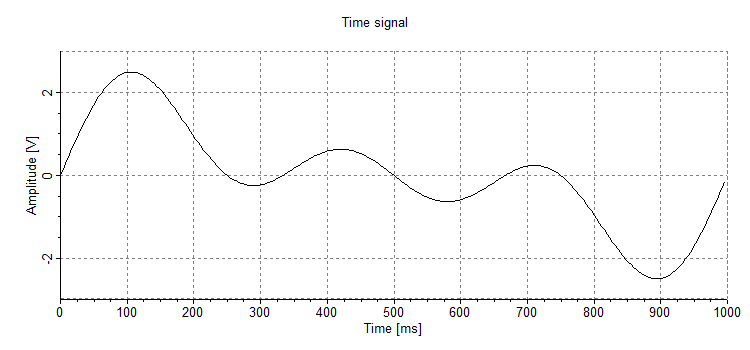And if we would like to extract the information of sin(1), we can apply the scalar product. We multiply this series with the sin that is of our interest.

Series(sin(1))*Series(sin1(1)    +   sin(2)   +    sin(3)) =

Series(sin(1))* Series(sin(1))    +    Series(sin(1))* Series(sin(2))   +   Series(sin(1))* Series(sin(3)) =

The second and the third parts are zero (in the infinity), because the arguments of the sines are different and therefore the two series are orthogonal and the scalar product is zero. Therefore:

Series(sin(1))*Series(sin1(1) + sin(2) + sin(3))  =  Series(sin(1))*Series(sin(1)) <> 0

This is how DFT extracts information about the frequency of the sines. If the applied series (sin(1)) has amplitude 1 then the resulting amplitude is exactly the amplitude of the sine searched for.  This is the second trick. Normally we would have to generate sines of all possible phases, frequencies and amplitudes to find the right one. We now generate only all possible frequencies and get the information of amplitude also if the sines are in-phase or aligned. (start at zero for example ). Phase aligned sines: (the red one is searched for and the black one is fitted):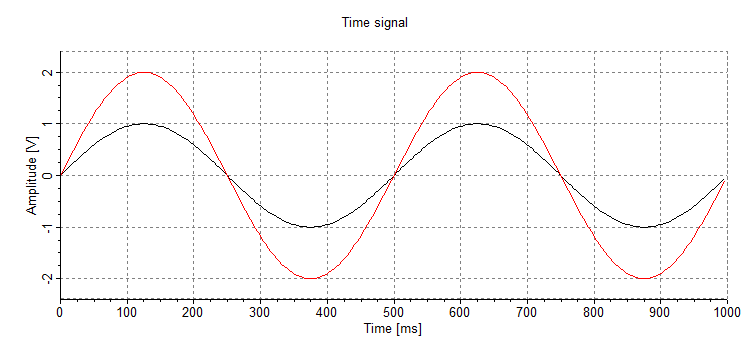If the sines were not phase aligned this would not work.

## The amplitude and the phase

So far we have been looking only at the frequency. The sines have two more parameters: amplitude and phase. A very neat trick (number 3) has been used to extract those two parameters efficiently. Phase alignment can be achieved by using a pair of fitting functions: sin and cos. These two functions have a neat property: sin2(x) + cos2(x)=1. and secondly, they are shifted by 90 degrees. Meaning: If we miss the sine for which we search with the fitting sine for 90 degrees, we hit exactly on the peak with the cosine. And in between we still have the property: sin2(x) + cos2(x)=1.  This formula can be thought of as calculating the amplitude of a vector whose projections on the real and imaginary axis are known. All we have to do is:

• multiply the time series with sines of all the possible frequencies, phase = 0 and Amplitude 1  (we get the real part of the frequency spectrum) and
• Multiply the time series with cosines of all the possible frequencies, phase = 0 and Amplitude = 1. (We get the imaginary part of the frequency spectrum.)

And here is where the Eulers notation comes in: e = cos(ω) +sin(ω). The first part is the real part and second part is the imaginary one. And the DFT can be written as:X[k] is of course complex and containes amplitudes at frequencies k and there is also no reason why x[n] couldn't be complex to. x[n] is the series that we analyze. And the argument: (2π / N- kn only controls the frequency of the sine and cosine. And finally we can write:Calculate the amplitude as: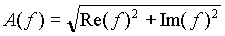, where f is the frequency for which we calculate the amplitude.

And the phase is calculated as:## The FFT

Physically nothing new. "Just" improvement of the calculation speed.

## Conclusion

The two main principles used in the derivation of the DFT are:

• Orthogonality of sines. (and cosines). Allows the extraction of a single sine from a sum of sines. The concept is widely used in linear algebra.
• The pair of sine and cosine. Computationally very efficient. Instead of fitting a large number of sines with different phase for each frequency, we fit only one sine and one cosine per frequency. If this concept wouldn't be known, there would be no DFT. Because of it, the accuracy of amplitude and phase estimation is way better than anybody could ever dream off with only a fraction of computational complexity.

It is clear now why Discrete Laplace transform could cause problems. We should fit the following function:The nice rules of DFT fall down and the number of parameters that we would need to control becomes very large. We only had to fit all the possible frequencies with DFT, but now at least one more parameter is required: attenuation. And we are already at computational complexity of at least O(n^3).

We can also start doubting the sines again. It has been done. The alternative is called Wavelets. Wavelets are also orthogonal functions. They are used in much the same way as sines. Unfortunately, wavelets are far from being natural. It is thought that this is their strength, but lack of physical interpretation is not a plus (not in physics anyway). Wavelets are not based so much on physics, like the DFT is.